Disclaimer: This is an example of a student written essay.

Any scientific information contained within this essay should not be treated as fact, this content is to be used for educational purposes only and may contain factual inaccuracies or be out of date.

# Weak Gravitational Lensing

 ✅ Paper Type: Free Essay ✅ Subject: Physics ✅ Wordcount: 2091 words ✅ Published: 26th Jul 2021

## Introduction

Gravitational lensing is a very resourceful phenomenon that widely used in astronomy measurement. It contains many aspects of which the strong, weak, and microlensing are well established by observable evidence. The weak gravitational lensing is an active area. Weak gravitational lensing that we can observe is usually caused by large-scale cosmic bodies such as clusters and voids. Generally, gravitational lensing has the advantage of directly measuring or comparing the mass of the object. This is an advantage comparing with the conventional method such as dynamical methods and the diffuse X-ray emission. Those methods require strong assumptions of either dynamical state or composition (Bartelmann & Schneider 2001). Thus, weak gravitational lensing has the prospect of leading us to understand more about the voids and clusters that other regular measurement could not achieve, which can further lead to verifying the existence and possible composition of dark matter.

## Method (How to measure & calculate)

Gravitational Lensing Effect

Gravitational lensing is the phenomenon that the light bundles bent when traveling by massive cosmic bodies due to the effect of gravitational force. In astrophysics photos, strong gravitational lensing would result in prominent arc or arclets (smaller arcs). However, weak gravitational lensing is relatively less noticeable since that the phenomenon only slightly distort the background galaxies. This distortion can be divided into two: convergence and shear. The convergence would result in magnification of the background while the shear would change the ellipticity of the background galaxies we observe.

If you need assistance with writing your essay, our professional essay writing service is here to help!

We show a simplistic example for weak gravitational lensing as Fig.1 shows. One thing to mention is that we used thin lens approximation for the example. This means that we assume the lensing effect take place in a surface, which is usually a good approximation because the distance from the background to the foreground ‘lens’ and from foreground ‘lens’ to observer are much long comparing the size of a cluster or void.

Considering a deflection caused by a point mass M. When a bundle of light pass through the weak gravitational field near it. The deflection angle predicted by general relativity is twice the amount of Newtonian predictionwhere impact factor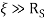(Schwarzschild radius), since the gravitational field is relatively small. Now considering that in Fig.1,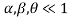, which is valid for most weak gravitational lensing application, it can be proven that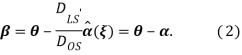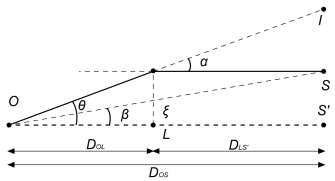Fig.1 An illustration of a typical gravitational lensing with S the source, O the observer and L the massive object

The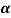above can be defined as scaled deflection angle, which can also be represented by surface density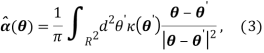where the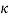denotes convergence and can be represented by surface mass density and critical surface density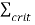: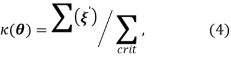with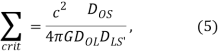where the distance is angular diameter distance. The critical surface density depends on the redshift of source and lens (Bartelmann & Schneider 2001), the convergence () represents the strength of the lensing. Generally,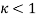represents weak lensing. Eq. (3) indicates that the deflection angle can also be written as the gradient of the deflection potential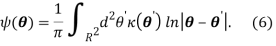As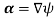, it can be prove that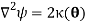The gravitational lensing doesn’t change the frequency of the photons, the deflection angles are all the same for any frequency (i.e. no dispersion). Considering that no photon has been emitted or absorbed and omit the change of gravitational potential over time, Liouville’s theorem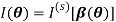Since the lens map can be linearized in weak gravitational field, the distortion of images is then described by the Jacobian matrix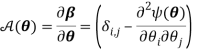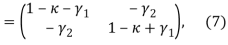where we need to introduced the shear components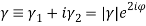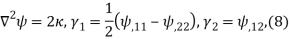Magnification factor in respect to fluxes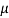can be represented by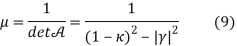In case of application, the reduced gravitational shear is more commonly used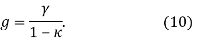(Bartelmann & Schneider 2001)

Weak Lensing by Voids

In Fig.1, if we assume L’ is the centre of a void, instead, then the figure can illustrate the example for weak lensing in a void. Note that weak lensing caused by clusters act like a ‘convex lens’, where it converge the light coming through, while those caused by voids are like ‘concave lens’, where the light is diverged. Though technically, they do not work the same as classical lensing since they do not have foci. This is because that the voids are and underdense and it is actually the mass outside the void that bend the light. Considering the fact that voids are also relatively large, the change of gravity potential along radius would not be as fast as in massive clusters. The weak lensing caused by voids are quite hard to detect (Amendola et al. 1999).

Another essential thing is to have a general model for voids. Due to the mass distribution of void, we shall assume it is a uniform spherical underdensity surrounded by overdense shell. While this is mere an ideal assumption, this model can still capture the main features for the voids (Amendola et al. 1999).

Implications

Clowe (2006) has put a convincing result with the help of weak gravitational lensing to indicate the existence of dark matter. During the collision of two clusters, they compare the mass of the plasma and of the whole clusters. The plasma was the dominant baryonic component. However, when they compared plasma distribution in X-ray and the cluster gravitational potential by weak lensing, the ratio of plasma/whole cluster fitted better in the cluster model with the dark matter.

More recent research have shown thatâ€¦

As we have mentioned that void is harder to detect due to its size and underdensity. In practice, the size measurement of an individual void in galaxy redshift surveys will be very noisy due to sparse sampling in the underdense region. That is one of the reasons why we need to get the void stacked to determine the weak lensing. Theoretical calculations by Krause et al. (2012) and numerical simulations by Higuchi, Oguri & Hamana (2013) suggest that stacking many voids will increase the signal-to-noise ratio (S/N) of the lensing observables, which may result in the detection of the effect with high significance, the lensing signals measured by stacking large numbers of voids with similar sizes will also constrain the radial profile of voids.

It is worthwhile to mention that the stacked method was first used in detection of weak lensing caused by clusters. However, with the improvement of astronomical imaging, the weak lensing for an individual cluster is much easier to detect.

Melchior et al. (2014) used the method to stack 901 voids detected in Sloan Digital Sky Survey (SSDS) DR7. They combined the voids with the coordinate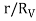, where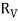is the radius of individual void. Their research indicates that voids are generally self-similar and that large voids may does not fit compensated void model as smaller voids do. This means that the density around large void, even for a sufficient large radius beyond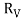, may still be lower than the average density.

Clampitt & Jain (2015) further extend the stacking method. They used a special method to identify the voids and then filter to remain the ones with good quality. They estimated S/N to be 7, which is a relatively high rate for void lensing. They gave a model-independent statement of void properties.

Limitations

One intrinsic problem for weak lensing is ‘ellipticity noise’. It denotes the fact that each background galaxy has its own ellipticity and orientation. The ‘ellipticity noise’ is often of the same level of the distortion or even larger (Bartelmann & Schneider 2001). Another key limitation of the gravitational lensing methodology could be that it only produces a two-dimensional map of k and hence raises the possibility that structures seen in the map are caused by physically unrelated masses along the line of sight (Clowe et al. 2006).

Reference

• Amendola, L., Frieman, J. A., & Waga, I. (1999). Weak gravitational lensing by voids. Monthly Notices of the Royal Astronomical Society, 309(2), 465-473.
• Bartelmann, M., & Schneider, P. (2001). Weak gravitational lensing. Physics Reports, 340(4), 291-472.
• Clampitt, J., & Jain, B. (2015). Lensing measurements of the mass distribution in SDSS voids. Monthly Notices of the Royal Astronomical Society, 454(4), 3357-3365.
• Clowe, D., BradaÄ, M., Gonzalez, A. H., Markevitch, M., Randall, S. W., Jones, C., & Zaritsky, D. (2006). A direct empirical proof of the existence of dark matter. The Astrophysical Journal Letters, 648(2), L109.
• Higuchi, Y., Oguri, M., & Hamana, T. (2013). Measuring the mass distribution of voids with stacked weak lensing. Monthly Notices of the Royal Astronomical Society, 432(2), 1021-1031.
• Kaiser, N., & Squires, G. (1993). Mapping the dark matter with weak gravitational lensing. The Astrophysical Journal, 404, 441-450.
• Krause, E., Chang, T. C., Doré, O., & Umetsu, K. (2012). The weight of emptiness: the gravitational lensing signal of stacked voids. The Astrophysical Journal Letters, 762(2), L20.
• Melchior, P., Sutter, P. M., Sheldon, E. S., Krause, E., & Wandelt, B. D. (2014). First measurement of gravitational lensing by cosmic voids in SDSS. Monthly Notices of the Royal Astronomical Society, 440(4), 2922-2927.

View all

## DMCA / Removal Request

If you are the original writer of this essay and no longer wish to have your work published on UKEssays.com then please:

Related Services

Prices from

SR571

Approximate costs for:

• 1000 words
• 7 day deliveryHumanity University

Dedicated to your worth and value as a human being!

Related Lectures